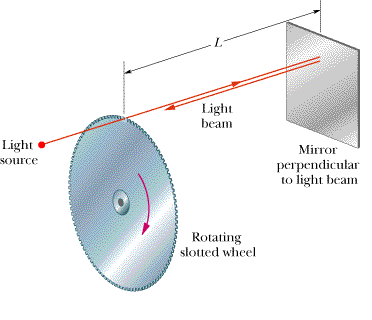# Physics Question Involving Angular Speed and Angular Velocity.

McAfee

## Homework Statement

An early method of measuring the speed of light makes use of a rotating slotted wheel. A beam of light passes through a slot at the outside edge of the wheel, as in Fig. 10-29, travels to a distant mirror, and returns to the wheel just in time to pass through the next slot in the wheel. One such slotted wheel has a radius of 5.6 cm and 75 slots at its edge. Measurements taken when the mirror is L = 800 m from the wheel indicate a speed of light of 3.0 x 105 km/s. (a) What is the (constant) angular speed of the wheel? (b) What is the linear speed of a point on the edge of the wheel?## Homework Equations

t=(2L)/C

angular velcity= answer is part a *.056m

## The Attempt at a Solution

For part (a)
I did use =angle/time

angle I solve (2Pi)/75 = .083776

I used t = 2L/c = 2(800)/(3x10^8) = .000005 sec

Then divided .083776/.000005 = 16755.2 rad/s

For part (b)

= my answer is part(a) * .056m

I know I'm doing something wrong, but don't know what it is. Can anyone help?

Staff Emeritus